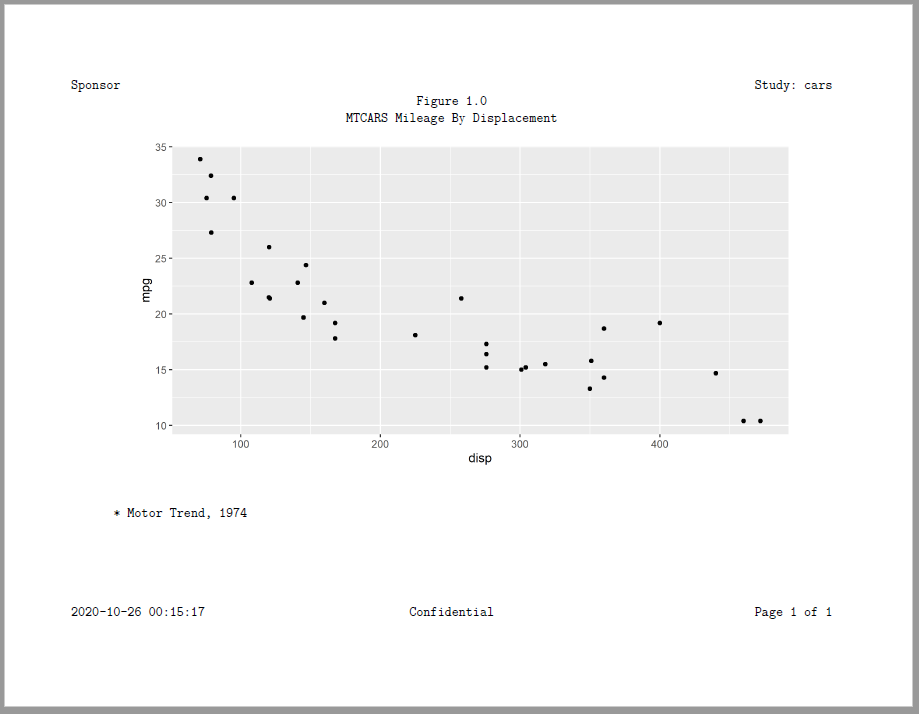### Create a Report with a Figure

RTF, PDF, HTML, and DOCX also support plots produced with the ggplot2 package. Use the create_plot() function to create plot content for a report. The plot can be added to the report with the add_content() function, just like tables and text.

If you want to add a custom image from another charting package, you may also pass a path to a JPEG file on the create_plot() function. The write_report() function will then get the image and insert it into the report at the specified location.

library(reporter)
library(ggplot2)

# Create temporary path
tmp <- file.path(tempdir(), "example10.pdf")

# Prepare data
dat <- mtcars[order(mtcars\$cyl), ]

# Generate plot
p <- ggplot(dat, aes(x=disp, y=mpg)) + geom_point()

# Define plot object
plt <- create_plot(p, height = 4, width = 8) %>%
titles("Figure 1.0", "MTCARS Mileage By Displacement", blank_row = "none") %>%
footnotes("* Motor Trend, 1974")

rpt <- create_report(tmp, output_type = "PDF") %>%
set_margins(top = 1, bottom = 1) %>%
options_fixed(font_size = 12) %>%
# file.show(tmp)